Do you like the article?
Share it with others -

Use new possibilities of MetaTrader 5

# Applying network functions, or MySQL without DLL: Part II - Program for monitoring changes in signal properties

14 May 2020, 11:08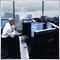0
10 433

### Introduction

In the previous part, we considered the implementation of the MySQL connector. Now it is time to consider the examples of its application. The simplest and most obvious one is collection of signal property values with the ability to view their further changes. Over 100 signals are available in the terminal for most accounts, while each signal has more than 20 parameters. This means we are going to have sufficient data. The implemented example has practical sense if users need to observe changes in properties that are not displayed on the signal's web page. These changes may include leverage, rating, number of subscribers and much more.

To collect data, let's implement the service that periodically requests for signal properties, compares them with previous values and sends the entire array to the database in case differences are detected.

To view the property dynamics, write an EA that displays a change in a selected property as a graph. Also, let's implement the ability to sort out signals by values of some properties using conditional database queries.

During the implementation, we will find out why it is desirable to use the Keep Alive constant connection mode and multiple queries in some cases.

### Data collection service

The service objectives are as follows:

1. Periodically request properties of all signals available in the terminal
2. Compare their values with the previous ones
3. If differences are detected, write the entire array of values to the database
4. Inform a user in case of errors

Create a new service in the editor and name it signals_to_db.mq5. The inputs are as follows:

```input string   inp_server     = "127.0.0.1";          // MySQL server address
input uint     inp_port       = 3306;                 // TCP port
input string   inp_db         = "signals_mt5";        // Database name
input bool     inp_creating   = true;                 // Allow creating tables
```

In addition to the network settings, there are several options here:

• inp_creating — permission to create tables in the database. If the service refers to a non-existing table, that table can be created if the parameter is equal to true
• inp_period — period of requesting signal properties in seconds

### Getting signal property values

In order for the service to work correctly, it is important to know when signal property values are updated in the terminal. This happens in two cases:
• During its launch.
• Periodically during terminal operation provided that the Signals tab in the Toolbox window is active. Data update periodicity is 3 hours.

At least, this is what happens in the terminal version as of the time of writing.

Signal properties we are interested in are of four types:

ENUM_SIGNAL_BASE_DATETIME type is created to detect the ENUM_SIGNAL_BASE_INTEGER properties that should be converted into the string as a time rather than an integer.

For convenience, decompose the enumeration values of same-type properties by arrays (four property types — four arrays). Each enumeration is accompanied by a text description of the property which is also the name of the appropriate field in the database table. Let's create the appropriate structures to achieve all this:

```//--- Structures of signal properties description for each type
struct STR_SIGNAL_BASE_DOUBLE
{
string                     name;
ENUM_SIGNAL_BASE_DOUBLE    id;
};
struct STR_SIGNAL_BASE_INTEGER
{
string                     name;
ENUM_SIGNAL_BASE_INTEGER   id;
};
struct STR_SIGNAL_BASE_DATETIME
{
string                     name;
ENUM_SIGNAL_BASE_INTEGER   id;
};
struct STR_SIGNAL_BASE_STRING
{
string                     name;
ENUM_SIGNAL_BASE_STRING    id;
};
```

Next, declare the structure arrays (below is an example for ENUM_SIGNAL_BASE_DOUBLE, it is similar for other types):

```const STR_SIGNAL_BASE_DOUBLE tab_signal_base_double[]=
{
{"Balance",    SIGNAL_BASE_BALANCE},
{"Equity",     SIGNAL_BASE_EQUITY},
{"Gain",       SIGNAL_BASE_GAIN},
{"Drawdown",   SIGNAL_BASE_MAX_DRAWDOWN},
{"Price",      SIGNAL_BASE_PRICE},
{"ROI",        SIGNAL_BASE_ROI}
};
```
Now, in order to receive the values of selected signal properties, we only need to move along the four loops:
```   //--- Read signal properties
{
for(int i=0; i<6; i++)
props_double[i] = SignalBaseGetDouble(ENUM_SIGNAL_BASE_DOUBLE(tab_signal_base_double[i].id));
for(int i=0; i<7; i++)
props_int[i] = SignalBaseGetInteger(ENUM_SIGNAL_BASE_INTEGER(tab_signal_base_integer[i].id));
for(int i=0; i<3; i++)
props_datetime[i] = datetime(SignalBaseGetInteger(ENUM_SIGNAL_BASE_INTEGER(tab_signal_base_datetime[i].id)));
for(int i=0; i<5; i++)
props_str[i] = SignalBaseGetString(ENUM_SIGNAL_BASE_STRING(tab_signal_base_string[i].id));
}
```

In the above example, Read() is a method of the SignalProperties structure featuring all you need to work with signal properties. These are the buffers for each of the types, as well as the methods for reading and comparing the current values with the previous ones:

```//--- Structure for working with signal properties
struct SignalProperties
{
//--- Property buffers
double            props_double;
long              props_int;
datetime          props_datetime;
string            props_str;
{
for(int i=0; i<6; i++)
props_double[i] = SignalBaseGetDouble(ENUM_SIGNAL_BASE_DOUBLE(tab_signal_base_double[i].id));
for(int i=0; i<7; i++)
props_int[i] = SignalBaseGetInteger(ENUM_SIGNAL_BASE_INTEGER(tab_signal_base_integer[i].id));
for(int i=0; i<3; i++)
props_datetime[i] = datetime(SignalBaseGetInteger(ENUM_SIGNAL_BASE_INTEGER(tab_signal_base_datetime[i].id)));
for(int i=0; i<5; i++)
props_str[i] = SignalBaseGetString(ENUM_SIGNAL_BASE_STRING(tab_signal_base_string[i].id));
}
//--- Compare signal Id with the passed value
bool              CompareId(long id)
{
if(id==props_int)
return true;
else
return false;
}
//--- Compare signal property values with the ones passed via the link
bool              Compare(SignalProperties &sig)
{
for(int i=0; i<6; i++)
{
if(props_double[i]!=sig.props_double[i])
return false;
}
for(int i=0; i<7; i++)
{
if(props_int[i]!=sig.props_int[i])
return false;
}
for(int i=0; i<3; i++)
{
if(props_datetime[i]!=sig.props_datetime[i])
return false;
}
return true;
}
//--- Compare signal property values with the one located inside the passed buffer (search by Id)
bool              Compare(SignalProperties &buf[])
{
int n = ArraySize(buf);
for(int i=0; i<n; i++)
{
if(props_int==buf[i].props_int)  // Id
return Compare(buf[i]);
}
return false;
}
};
```

To work with the database, we first need to declare the instance of the CMySQLTransaction class:

```//--- Include MySQL transaction class
#include  <MySQL\MySQLTransaction.mqh>
CMySQLTransaction mysqlt;
```

Next, set connection parameters in the OnStart() function. To do this, call the Config method:

```//+------------------------------------------------------------------+
//| Service program start function                                   |
//+------------------------------------------------------------------+
void OnStart()
{
//--- Configure MySQL transaction class

...
}
```

The next step is creating the table name. Since the set of signals depends on a broker and a trading account type, these parameters should be used to achieve this. In the server name, replace the period, hyphen and space with an underscore, add the account login and replace all letters with lowercase ones. For example, in case of the MetaQuotes-Demo broker server and the login of 17273508, the table name is metaquotes_demo__17273508.

This looks as follows in the code:

```//--- Assign a name to the table
//--- to do this, get the trade server name
string s = AccountInfoString(ACCOUNT_SERVER);
//--- replace the space, period and hyphen with underscores
string ss[]= {" ",".","-"};
for(int i=0; i<3; i++)
StringReplace(s,ss[i],"_");
//--- assemble the table name using the server name and the trading account login
//--- set all letters to lowercase
StringToLower(tab_name);
//--- display the result in the console
Print("Table name: ",tab_name);
```

Next, read the last entry data to the database. This is done so that it is possible to compare obtained properties with something to detect differences when restarting the service.

```//+------------------------------------------------------------------+
//| Read signal properties from the database                         |
//+------------------------------------------------------------------+
{
//--- prepare a query
string q="select * from `"+inp_db+"`.`"+tab_name+"` "+
"where `TimeInsert`= ("+
"select `TimeInsert` "+
"from `"+inp_db+"`.`"+tab_name+"` order by `TimeInsert` desc limit 1)";
//--- send a query
if(mysqlt.Query(q)==false)
return false;
//--- if the query is successful, get the pointer to it
CMySQLResponse *r = mysqlt.Response();
if(CheckPointer(r)==POINTER_INVALID)
return false;
//--- read the number of rows in the accepted response
uint rows = r.Rows();
//--- prepare the array
if(ArrayResize(sbuf,rows)!=rows)
return false;
//--- read property values to the array
for(uint n=0; n<rows; n++)
{
//--- read the pointer to the current row
CMySQLRow *row = r.Row(n);
if(CheckPointer(row)==POINTER_INVALID)
return false;
for(int i=0; i<6; i++)
{
if(row.Double(tab_signal_base_double[i].name,sbuf[n].props_double[i])==false)
return false;
}
for(int i=0; i<7; i++)
{
if(row.Long(tab_signal_base_integer[i].name,sbuf[n].props_int[i])==false)
return false;
}
for(int i=0; i<3; i++)
sbuf[n].props_datetime[i] = MySQLToDatetime(row[tab_signal_base_datetime[i].name]);
for(int i=0; i<5; i++)
sbuf[n].props_str[i] = row[tab_signal_base_string[i].name];
}
return true;
}
```
Function arguments are references to the signal buffer and table name we formed during initialization. The first thing we do in the function body is prepare a query. In this case, we need to read all the properties of the signals having the maximum time of adding to the database. Since we write the array of all values simultaneously, we need to find the maximum time in the table and read all the strings where the time of adding is equal to the found one. For example, if the database name is signals_mt5, while the table name is metaquotes_demo__17273508, the query will look as follows:
```select *
from `signals_mt5`.`metaquotes_demo__17273508`
where `TimeInsert`= (
select `TimeInsert`
from `signals_mt5`.`metaquotes_demo__17273508`
order by `TimeInsert` desc limit 1)
```

The subquery to return the maximum `TimeInsert` column value, i.e. the time of the last adding to the database, is highlighted in red. The query highlighted in green returns all strings where the `TimeInsert` value matches the found one.

If the transaction is successful, start reading obtained data. To do this, get the pointer to the CMySQLResponse server response class, then get the number of rows in the response. Depending on that parameter, change the signal buffer size.

Now we need to read the properties. To do this, receive the pointer to the current row using the index. After that, read the values for each property type. For example, to read the ENUM_SIGNAL_BASE_DOUBLE properties, use the CMySQLRow::Double() method where the first argument (field name) is a property text name.

Let's consider the case, in which sending a query ends in an error. To do this, return to the OnStart() function source code.
```//--- Declare the buffer of signal properties
SignalProperties sbuf[];
//--- Raise the data from the database to the buffer
bool exit = false;
{
//--- if the reading function returns an error,
//--- the table is possibly missing
if(mysqlt.GetServerError().code==ER_NO_SUCH_TABLE && inp_creating==true)
{
//--- if we need to create a table and this is allowed in the settings
if(DB_CteateTable(tab_name)==false)
exit=true;
}
else
exit=true;
}
```

In case of an error, first of all, check if it is not caused by the table absence. This error occurs if the table is not created yet, removed or renamed. The ER_NO_SUCH_TABLE error means the table should be created (if allowed).

The DB_CteateTable() table creation function is quite simple:

```//+------------------------------------------------------------------+
//| Create the table                                                 |
//+------------------------------------------------------------------+
bool  DB_CteateTable(string name)
{
//--- prepare a query
string q="CREATE TABLE `"+inp_db+"`.`"+name+"` ("+
"`PKey`                        BIGINT(20)   NOT NULL AUTO_INCREMENT,"+
"`TimeInsert`     DATETIME    NOT NULL,"+
"`Id`             INT(11)     NOT NULL,"+
"`Name`           CHAR(50)    NOT NULL,"+
"`Broker`         CHAR(50)    NOT NULL,"+
"`BrokerServer`   CHAR(50)    NOT NULL,"+
"`Balance`        DOUBLE      NOT NULL,"+
"`Equity`         DOUBLE      NOT NULL,"+
"`Gain`           DOUBLE      NOT NULL,"+
"`Drawdown`       DOUBLE      NOT NULL,"+
"`Price`          DOUBLE      NOT NULL,"+
"`ROI`            DOUBLE      NOT NULL,"+
"`Leverage`       INT(11)     NOT NULL,"+
"`Pips`           INT(11)     NOT NULL,"+
"`Rating`         INT(11)     NOT NULL,"+
"`Subscribers`    INT(11)     NOT NULL,"+
"`Published`      DATETIME    NOT NULL,"+
"`Started`        DATETIME    NOT NULL,"+
"`Updated`        DATETIME    NOT NULL,"+
"`Currency`       CHAR(50)    NOT NULL,"+
"PRIMARY KEY (`PKey`),"+
"UNIQUE INDEX `TimeInsert_Id` (`TimeInsert`, `Id`),"+
"INDEX `TimeInsert` (`TimeInsert`),"+
"INDEX `Currency` (`Currency`, `TimeInsert`),"+
"INDEX `Broker` (`Broker`, `TimeInsert`),"+
"INDEX `Id` (`Id`, `TimeInsert`)"+
") COLLATE='utf8_general_ci' "+
"ENGINE=InnoDB "+
"ROW_FORMAT=DYNAMIC";
//--- send a query
if(mysqlt.Query(q)==false)
return false;
return true;
}
```
The query itself features the time of adding `TimeInsert` data among the field names which are names of signal properties. This is the local terminal time at the moment of receiving the updated properties. Besides, there is a unique key for the `TimeInsert` and `Id` fields, as well as indices necessary for accelerating query execution.

If the table creation fails, display the error description and terminate the service.

```   if(exit==true)
{
if(GetLastError()==(ERR_USER_ERROR_FIRST+MYSQL_ERR_SERVER_ERROR))
{
// in case of a server error
Print("MySQL Server Error: ",mysqlt.GetServerError().code," (",mysqlt.GetServerError().message,")");
}
else
{
if(GetLastError()>=ERR_USER_ERROR_FIRST)
Print("Transaction Error: ",EnumToString(ENUM_TRANSACTION_ERROR(GetLastError()-ERR_USER_ERROR_FIRST)));
else
Print("Error: ",GetLastError());
}
return;
}
```

We can have three kinds of errors.

• The error returned by the MySQL server (no table, no database, invalid login or password)
• The runtime error (invalid host, connection error)
• The ENUM_TRANSACTION_ERROR error

The error type defines how its description is formed. The error is defined as follows.

If the transaction passes without errors, we enter the main program loop:

```//--- set the time label of the previous reading of signal properties
datetime chk_ts = 0;

...
//--- Main loop of the service operation
do
{
if((TimeLocal()-chk_ts)<inp_period)
{
Sleep(1000);
continue;
}
//--- it is time to read signal properties
chk_ts = TimeLocal();

...

}
while(!IsStopped());
```

Our service is to be located in this endless loop till it is unloaded. The properties are read, comparisons with the previous values are performed and writing to the database is done (if necessary) with a specified periodicity.

Suppose that we have received signal properties that differ from the previous values. The following happens next:

```      if(newdata==true)
{
bool bypass = false;
if(DB_Write(buf,tab_name,chk_ts)==false)
{
//--- if we need to create a table and this is allowed in the settings
if(mysqlt.GetServerError().code==ER_NO_SUCH_TABLE && inp_creating==true)
{
if(DB_CteateTable(tab_name)==true)
{
//--- if the table is created successfully, send the data
if(DB_Write(buf,tab_name,chk_ts)==false)
bypass = true; // sending failed
}
else
bypass = true; // failed to create the table
}
else
bypass = true; // there is no table and it is not allowed to create one
}
if(bypass==true)
{
if(GetLastError()==(ERR_USER_ERROR_FIRST+MYSQL_ERR_SERVER_ERROR))
{
// in case of a server error
PrintNotify("MySQL Server Error: "+IntegerToString(mysqlt.GetServerError().code)+" ("+mysqlt.GetServerError().message+")");
}
else
{
if(GetLastError()>=ERR_USER_ERROR_FIRST)
PrintNotify("Transaction Error: "+EnumToString(ENUM_TRANSACTION_ERROR(GetLastError()-ERR_USER_ERROR_FIRST)));
else
PrintNotify("Error: "+IntegerToString(GetLastError()));
}
continue;
}
}
else
continue;
```

Here we can see the familiar code fragment featuring the check for the table absence and its subsequent creation. This enables correct handling of the table deletion by a third party while the service is running. Also, note that Print() is replaced with PrintNotify(). This function duplicates the string displayed in the console as a notification if this is allowed in the inputs:

```//+------------------------------------------------------------------+
//| Print to console and send notification                           |
//+------------------------------------------------------------------+
void PrintNotify(string text)
{
//--- display in the console
Print(text);
{
static datetime ts = 0;       // last notification sending time
static string prev_text = ""; // last notification text
if(text!=prev_text || (text==prev_text && (TimeLocal()-ts)>=(3600*6)))
{
// identical notifications are sent one after another no more than once every 6 hours
{
ts = TimeLocal();
prev_text = text;
}
}
}
}
```

When detecting property updates, call the function for writing to the database:

```//+------------------------------------------------------------------+
//| Write signal properties to the database                          |
//+------------------------------------------------------------------+
bool  DB_Write(SignalProperties &sbuf[],string tab_name,datetime tc)
{
//--- prepare a query
string q = "insert ignore into `"+inp_db+"`.`"+tab_name+"` (";
q+= "`TimeInsert`";
for(int i=0; i<6; i++)
q+= ",`"+tab_signal_base_double[i].name+"`";
for(int i=0; i<7; i++)
q+= ",`"+tab_signal_base_integer[i].name+"`";
for(int i=0; i<3; i++)
q+= ",`"+tab_signal_base_datetime[i].name+"`";
for(int i=0; i<5; i++)
q+= ",`"+tab_signal_base_string[i].name+"`";
q+= ") values ";
int sz = ArraySize(sbuf);
for(int s=0; s<sz; s++)
{
q+=(s==0)?"(":",(";
q+= "'"+DatetimeToMySQL(tc)+"'";
for(int i=0; i<6; i++)
q+= ",'"+DoubleToString(sbuf[s].props_double[i],4)+"'";
for(int i=0; i<7; i++)
q+= ",'"+IntegerToString(sbuf[s].props_int[i])+"'";
for(int i=0; i<3; i++)
q+= ",'"+DatetimeToMySQL(sbuf[s].props_datetime[i])+"'";
for(int i=0; i<5; i++)
q+= ",'"+sbuf[s].props_str[i]+"'";
q+=")";
}
//--- send a query
if(mysqlt.Query(q)==false)
return false;
//--- if the query is successful, get the pointer to it
CMySQLResponse *r = mysqlt.Response(0);
if(CheckPointer(r)==POINTER_INVALID)
return false;
//--- the Ok type packet should be received as a response featuring the number of affected rows; display it
if(r.Type()==MYSQL_RESPONSE_OK)
//
return true;
}
```

Traditionally, the function code begins with a query formation. Due to the fact that we decomposed same-type properties into arrays, obtaining the list of fields and values is performed in loops and looks very compact in the code.

After sending a query, we expect the Ok type response from the server. From that response, we obtain the number of affected rows using the AffectedRows() method. That number is displayed in the console. In case of a failure, the function returns false entailing the error message being displayed in the console and sent as a notification if this is allowed in the settings. The obtained properties are not copied to the main buffer. After the specified period, a new change of their values is detected and an attempt to write them to the database is made.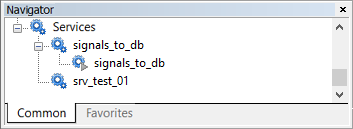Fig. 1. Launched signal properties collection service

Fig. 1 displays the launched signals_to_db service as it is seen in the Navigator window. Do not forget about selecting the Signals tab as mentioned above, otherwise the service will not receive new data.

### Application for viewing property dynamics

In the previous section, we implemented the service adding to the database the values of signal properties when a change is detected. The next step is preparing an application meant for displaying the selected property dynamics within a specified time interval as a graph. It will also be possible to select only the signals we are interested in using the filters of certain property values.

Since the application is to have an advanced GUI, EasyAndFastGUI library for creating graphical interfaces by Anatoli Kazharski is to be used as a basis.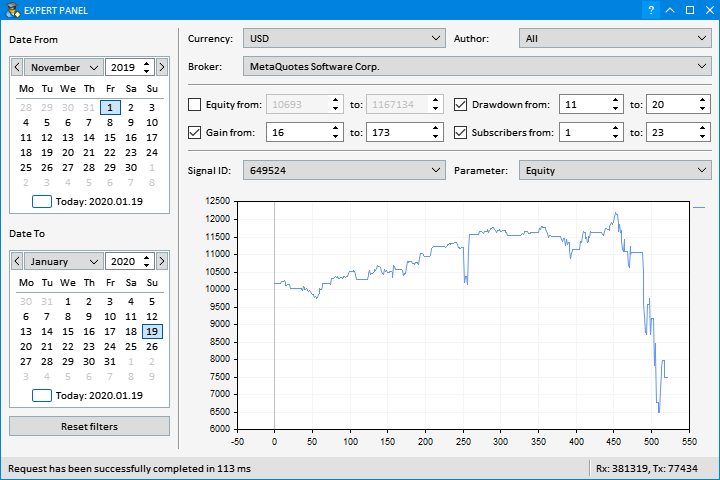a)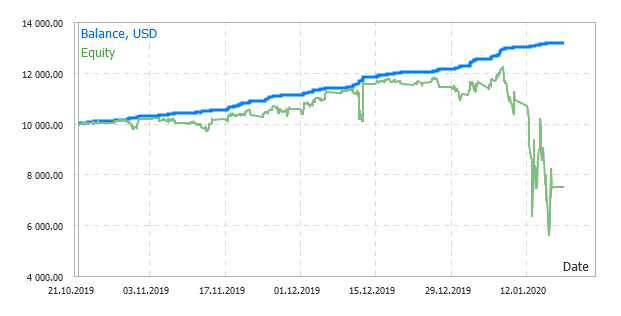b)

Fig. 2. The program user interface: the selected signal's Equity dynamics graph (a); the same graph on the signal's web page (b)

Fig. 2a features the look of the program user interface. The left part contains the date range, while the right one has the graph of Equity property of the selected signal. For comparison, Fig. 2b features the screenshot of a web page of the signal with the Equity property graph. The reason for the small discrepancies lies in the database "holes" formed during the PC idle time, as well as the relatively large period of updating the values of the signal properties in the terminal.

So, let the application have the following functionality:

• When selecting data from the table, it is able to:
• Set a date range
• Set a condition by SIGNAL_BASE_CURRENCY, SIGNAL_BASE_AUTHOR_LOGIN and SIGNAL_BASE_BROKER property values
• Set the range of valid values for the SIGNAL_BASE_EQUITY, SIGNAL_BASE_GAIN, SIGNAL_BASE_MAX_DRAWDOWN and SIGNAL_BASE_SUBSCRIBERS properties
• Construct the graph of a specified property when selecting the SIGNAL_BASE_ID value

### Implementation

Out of all graphical elements, we need a block of two calendars to set "from" and "to" dates, a group of combo boxes to select values from lists and the block of input fields to edit extreme property values in case a range should be set. To disable the conditions, use the "All" key value for lists, which is located at the very beginning. Also, equip the input field blocks with a checkbox which is disabled by default.

The date range should be specified at all times. Everything else can be customized as needed. Fig. 2a shows that a currency and a broker are set rigidly in the string property block, while a signal author's name is not regulated (All).

Each combo box list is formed using data obtained when handling a query. This is also true for input fields' extreme values. After forming the list of signal IDs and selecting some of its elements, the query for data to plot a graph of a specified property is sent.

To have more info on how the program interacts with the MySQL server, display the counters of accepted and sent bytes, as well as the last transaction time (Fig. 2) in the status bar. If a transaction fails, display the error code (Fig. 3).

###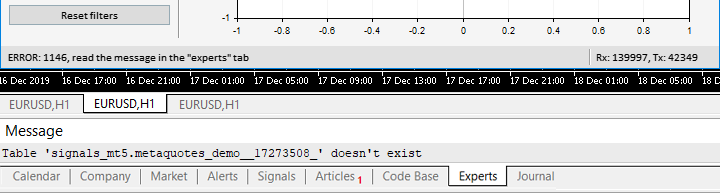Fig. 3. Displaying the error code in the status bar and the message in the Experts tab

Since most textual descriptions of server errors do not fit into the progress bar, display them in the Experts tab.

Since the current article has nothing to do with graphics, I am not going to dwell on implementing the user interface here. Working with the library is described in detail by its author in the series of articles. I have made some changes in a few files of the example taken as the basis, namely:

• MainWindow.mqh — building a graphical interface
• Program.mqh — interacting with the graphical interface
• Main.mqh — working with the database (added)

### Multiple queries

Database queries used when running the program can be roughly divided into three groups:

• Queries for obtaining combo box list values
• Queries for obtaining extreme values of input field blocks
• Queries for data to build a graph

While in the latter two cases, a single SELECT query is sufficient, the former one requires sending a separate query for each of the lists. At the some time, we cannot increase the time for obtaining data. Ideally, all values should be updated simultaneously. Also, it is impossible to update only a part of lists. To do this, use a multiple query. Even if a transaction (including handling and transfer) is delayed, the interface is updated only after all server responses are accepted. In case of an error, the partial update of the lists of interface graphical elements is disabled.

Below is a sample multiple query sent immediately when launching the program.

```select `Currency` from `signals_mt5`.`metaquotes_demo__17273508`
where `TimeInsert`>='2019-11-01 00:00:00' AND `TimeInsert`<='2019-12-15 23:59:59'
group by `Currency`;
select `Broker` from `signals_mt5`.`metaquotes_demo__17273508`
where `TimeInsert`>='2019-11-01 00:00:00' AND `TimeInsert`<='2019-12-15 23:59:59'
group by `Broker`;
where `TimeInsert`>='2019-11-01 00:00:00' AND `TimeInsert`<='2019-12-15 23:59:59'
select `Id` from `signals_mt5`.`metaquotes_demo__17273508`
where `TimeInsert`>='2019-11-01 00:00:00' AND `TimeInsert`<='2019-12-15 23:59:59'
group by `Id`;
select  Min(`Equity`) as EquityMin,             Max(`Equity`) as EquityMax,
Min(`Gain`) as GainMin,                 Max(`Gain`) as GainMax,
Min(`Drawdown`) as DrawdownMin,         Max(`Drawdown`) as DrawdownMax,
Min(`Subscribers`) as SubscribersMin,   Max(`Subscribers`) as SubscribersMax
from `signals_mt5`.`metaquotes_demo__17273508`
where `TimeInsert`>='2019-11-01 00:00:00' AND `TimeInsert`<='2019-12-15 23:59:59'
```

As we can see, this is a sequence of five "SELECT" queries separated by ";". The first four ones request the lists of unique values of specified properties (Currency, Broker, AuthorLogin and Id) in a specified time interval. The fifth query is designed to receive the minimum and maximum values of four properties (Equity, Gain, Drawdown and Subscribers) from the same time interval.

If we look at the data exchange with the MySQL server, we can see that: the query (1) was sent in a single TCP packet, while the responses to it (2) were delivered in different TCP packets (see Fig. 4).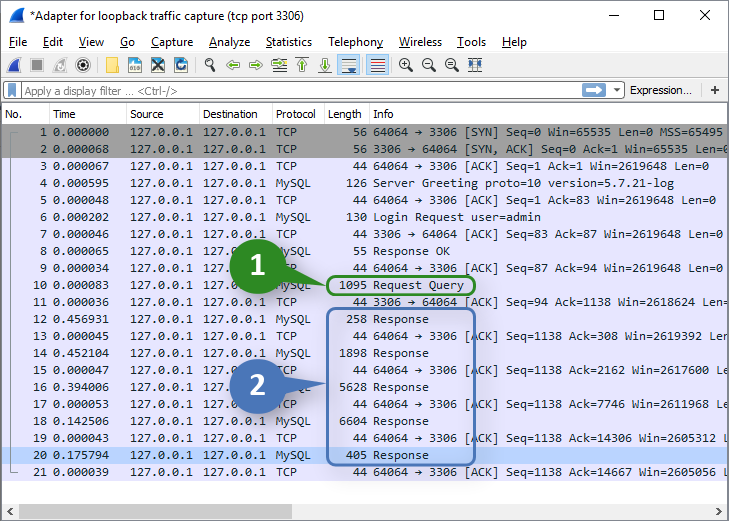Fig. 4. The multiple query in the traffic analyzer

Note that if one of the nested "SELECT" queries causes an error, the subsequent ones are not handled. In other words, the MySQL server handles queries up to the first error.

### Filters

For more convenience, let's add the filters reducing the list of signals leaving only the ones meeting the defined requirements. For example, we are interested in signals with a certain base currency, a specified growth range or a non-zero number of subscribers. To do this, apply the WHERE operator in the query:

```select `Broker` from `signals_mt5`.`metaquotes_demo__17273508`
where `TimeInsert`>='2019-11-01 00:00:00' AND `TimeInsert`<='2019-12-15 23:59:59' AND `Currency`='USD' AND `Gain`>='100' AND `Gain`<='1399'
group by `Broker`;
where `TimeInsert`>='2019-11-01 00:00:00' AND `TimeInsert`<='2019-12-15 23:59:59' AND `Currency`='USD' AND `Gain`>='100' AND `Gain`<='1399'
select `Id` from `signals_mt5`.`metaquotes_demo__17273508`
where `TimeInsert`>='2019-11-01 00:00:00' AND `TimeInsert`<='2019-12-15 23:59:59' AND `Currency`='USD' AND `Gain`>='100' AND `Gain`<='1399'
group by `Id`;
select  Min(`Equity`) as EquityMin,             Max(`Equity`) as EquityMax,
Min(`Gain`) as GainMin,                 Max(`Gain`) as GainMax,
Min(`Drawdown`) as DrawdownMin,         Max(`Drawdown`) as DrawdownMax,
Min(`Subscribers`) as SubscribersMin,   Max(`Subscribers`) as SubscribersMax
from `signals_mt5`.`metaquotes_demo__17273508`
where `TimeInsert`>='2019-11-01 00:00:00' AND `TimeInsert`<='2019-12-15 23:59:59' AND `Currency`='USD' AND `Gain`>='100' AND `Gain`<='1399'
```

The above query is intended for obtaining combo box lists and extreme values of input fields provided that the base currency is USD, while the growth value lies within the range of 100-1399. Here we need to first pay attention to the absence of a query for values from the Currency list. This is logical since we exclude all values while selecting a specific one in the combo box list. At the same time, the query for values is always performed for input fields even if they are used in the condition. This is done to let a user see a real range of values. Suppose that we have introduced the minimum growth value of 100. However, considering the data set meeting the selected criteria, the nearest minimum value is 135. This means that after receiving the server response, the value of 100 is replaced with 135.

After making a query with specified filters, the list of Signal ID combo box values is reduced significantly. It is possible to select a signal and track the changes of its properties on the graph.

### Keep Alive constant connection mode

If we look thoroughly at Fig. 4, we can see that there is no connection closure there. The reason for that is that the program for viewing signal property dynamics applies the constant connection mode we are to consider here.

When developing the data collection service, we left the "constant connection" parameter disabled. The data was rarely recorded and there was no point in keeping the connection. This is not the case here. Suppose that a user is looking for a suitable signal using the dynamics graph of a certain property. The query is sent to the database each time any of the control elements is modified. It would not be entirely correct to establish and close the connection every time in this case.

To activate the constant connection mode, set its timeout equal to 60 seconds.

```   if(CheckPointer(mysqlt)==POINTER_INVALID)
{
mysqlt = new CMySQLTransaction;
mysqlt.PingPeriod(10000);
}
```

This means, the connection is closed if a user remains idle for more than 60 seconds.

Let's see how this looks in practice. Suppose that a user has changed a certain parameter remaining idle for a minute after that. Capturing network packets will look as follows: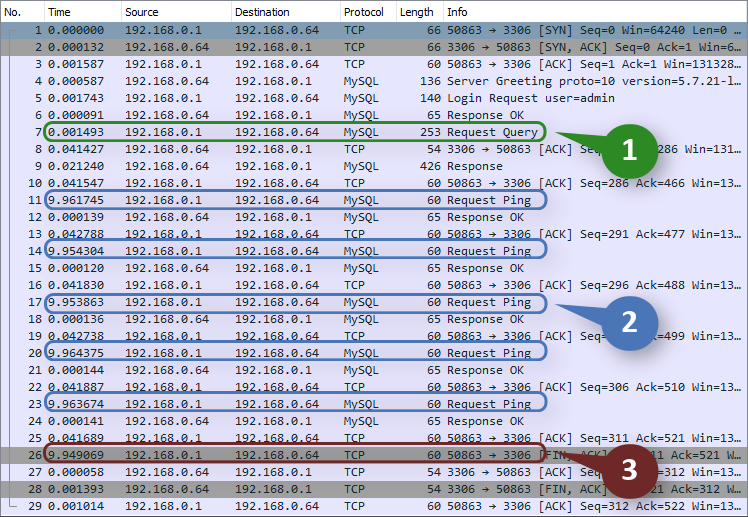Fig. 5. Capturing the packets when working in the Keep Alive mode

The image shows the query (1), the ping series with the period of 10 seconds (2) and closing the connection upon one minute passes after the query (3). If the user had continued the work and queries had been sent more often than once per minute, the connection would not have been closed.

Specifying transaction class parameters was also accompanied by the ping period equal to 10 seconds. Why do we need it? First of all, it is necessary so that the server does not close the connection from its side according to the timeout set in the configuration, provided that the timeout value can be obtained using the following query:

```show variables
where `Variable_name`='interactive_timeout'
```

Most often, it is 3 600 seconds. Theoretically, it is sufficient to send a ping with a period that is less than the server timeout, thus preventing the connection from being closed from its side. But in this case, we are able to know about the connection loss only when sending the next query. On the contrary, when the value of 10 seconds is set, we are able to know about the connection loss almost immediately.

### Data retrieval

Let's have a look at the server response to a multiple query using the GetData method implementation as an example. The method is designed for updating the content of drop-down lists, extreme values of input fields, as well as the dynamics graph of a selected property.
```void CMain::GetData(void)
{
if(CheckPointer(mysqlt)==POINTER_INVALID)
{
mysqlt = new CMySQLTransaction;
mysqlt.PingPeriod(10000);
}
//--- Save signal id
string signal_id = SignalId();
if(signal_id=="Select...")
signal_id="";
//--- Make a query
string   q = "";
if(Currency()=="All")
{
q+= "select `Currency` from `"+m_mysql_db+"`.`"+m_mysql_table+"` where "+Condition()+" group by `Currency`; ";
}
if(Broker()=="All")
{
q+= "select `Broker` from `"+m_mysql_db+"`.`"+m_mysql_table+"` where "+Condition()+" group by `Broker`; ";
}
if(Author()=="All")
{
}
q+= "select `Id` from `"+m_mysql_db+"`.`"+m_mysql_table+"` where "+Condition()+" group by `Id`; ";
q+= "select Min(`Equity`) as EquityMin, Max(`Equity`) as EquityMax";
q+= ", Min(`Gain`) as GainMin, Max(`Gain`) as GainMax";
q+= ", Min(`Drawdown`) as DrawdownMin, Max(`Drawdown`) as DrawdownMax";
q+= ", Min(`Subscribers`) as SubscribersMin, Max(`Subscribers`) as SubscribersMax from `"+m_mysql_db+"`.`"+m_mysql_table+"` where "+Condition();
//--- Display the transaction result in the status bar
return;
//--- Set accepted values in the combo box lists and extreme values of the input fields
uint responses = mysqlt.Responses();
for(uint j=0; j<responses; j++)
{
if(mysqlt.Response(j).Fields()<1)
continue;
if(UpdateComboBox(m_currency,mysqlt.Response(j),"Currency")==true)
continue;
if(UpdateComboBox(m_broker,mysqlt.Response(j),"Broker")==true)
continue;
continue;
if(UpdateComboBox(m_signal_id,mysqlt.Response(j),"Id",signal_id)==true)
continue;
//
UpdateTextEditRange(m_equity_from,m_equity_to,mysqlt.Response(j),"Equity");
UpdateTextEditRange(m_gain_from,m_gain_to,mysqlt.Response(j),"Gain");
UpdateTextEditRange(m_drawdown_from,m_drawdown_to,mysqlt.Response(j),"Drawdown");
UpdateTextEditRange(m_subscribers_from,m_subscribers_to,mysqlt.Response(j),"Subscribers");
}
GetSeries();
}
```

First, form a query. Regarding combo box lists, only the lists with the currently selected value of All are included to the query. Conditions are assembled in the separate Condition() method:

```string CMain::Condition(void)
{
string s = "`TimeInsert`>='"+time_from(TimeFrom())+"' AND `TimeInsert`<='"+time_to(TimeTo())+"' ";
//--- Add the remaining conditions if required
//--- For drop-down lists, the current value should not be equal to All
if(Currency()!="All")
s+= "AND `Currency`='"+Currency()+"' ";
if(Broker()!="All")
{
string broker = Broker();
//--- the names of some brokers contain characters that should be escaped
StringReplace(broker,"'","\\'");
s+= "AND `Broker`='"+broker+"' ";
}
if(Author()!="All")
//--- A checkbox should be set for input fields
if(m_equity_from.IsPressed()==true)
s+= "AND `Equity`>='"+m_equity_from.GetValue()+"' AND `Equity`<='"+m_equity_to.GetValue()+"' ";
if(m_gain_from.IsPressed()==true)
s+= "AND `Gain`>='"+m_gain_from.GetValue()+"' AND `Gain`<='"+m_gain_to.GetValue()+"' ";
if(m_drawdown_from.IsPressed()==true)
s+= "AND `Drawdown`>='"+m_drawdown_from.GetValue()+"' AND `Drawdown`<='"+m_drawdown_to.GetValue()+"' ";
if(m_subscribers_from.IsPressed()==true)
s+= "AND `Subscribers`>='"+m_subscribers_from.GetValue()+"' AND `Subscribers`<='"+m_subscribers_to.GetValue()+"' ";
return s;
}
```

If the transaction is successful, get the number of responses we then analyze in the loop.

The UpdateComboBox() method is designed for updating data in combo boxes. It receives a pointer to the response and the corresponding field name. If the field exists in the response, the data is included to the combo box list and the method returns true. The set_value argument contains the value from the previous list selected by the user during the query. It should be found in the new list and set as the current one. If the specified value is not present in the new list, the value under the index of 1 is set (following "Select...").

```bool CMain::UpdateComboBox(CComboBox &object, CMySQLResponse *p, string name, string set_value="")
{
int col_idx = p.Field(name);
if(col_idx<0)
return false;
uint total = p.Rows()+1;
if(total!=object.GetListViewPointer().ItemsTotal())
{
string tmp = object.GetListViewPointer().GetValue(0);
object.GetListViewPointer().Clear();
object.ItemsTotal(total);
object.SetValue(0,tmp);
object.GetListViewPointer().YSize(18*((total>16)?16:total)+3);
}
uint set_val_idx = 0;
for(uint i=1; i<total; i++)
{
string value = p.Value(i-1,col_idx);
object.SetValue(i,value);
if(set_value!="" && value==set_value)
set_val_idx = i;
}
//--- if there is no specified value, but there are others, select the topmost one
if(set_value!="" && set_val_idx==0 && total>1)
set_val_idx=1;
//---
ComboSelectItem(object,set_val_idx);
//---
return true;
}
```

The UpdateTextEditRange() method updates extreme values of text input fields.

```bool CMain::UpdateTextEditRange(CTextEdit &obj_from,CTextEdit &obj_to, CMySQLResponse *p, string name)
{
if(p.Rows()<1)
return false;
else
return SetTextEditRange(obj_from,obj_to,p.Value(0,name+"Min"),p.Value(0,name+"Max"));
}
```

Before exiting GetData(), the GetSeries() method is called which selects data by a signal ID and a property name:

```void CMain::GetSeries(void)
{
if(SignalId()=="Select...")
{
// if a signal is not selected
ArrayFree(x_buf);
ArrayFree(y_buf);
return;
}
if(CheckPointer(mysqlt)==POINTER_INVALID)
{
mysqlt = new CMySQLTransaction;
mysqlt.PingPeriod(10000);
}
string   q = "select `"+Parameter()+"` ";
q+= "from `"+m_mysql_db+"`.`"+m_mysql_table+"` ";
q+= "where `TimeInsert`>='"+time_from(TimeFrom())+"' AND `TimeInsert`<='"+time_to(TimeTo())+"' ";
q+= "AND `Id`='"+SignalId()+"' order by `TimeInsert` asc";

//--- Send a query
return;
//--- Check the number of responses
if(mysqlt.Responses()<1)
return;
CMySQLResponse *r = mysqlt.Response(0);
uint rows = r.Rows();
if(rows<1)
return;
//--- copy the column to the graph data buffer (false - do not check the types)
if(r.ColumnToArray(Parameter(),y_buf,false)<1)
return;
//--- form X axis labels
if(ArrayResize(x_buf,rows)!=rows)
return;
for(uint i=0; i<rows; i++)
x_buf[i] = i;
//--- Update the graph
}
```

Generally, its implementation is similar to the GetData() method discussed above. But there are two things worth paying attention to:

• If a signal is not selected (the combo box value is equal to "Select..."), the graph is cleared and nothing else happens.
• Using the ColumnToArray() method

The mentioned method is designed exactly for the cases when the column data should be copied to the buffer. In the current case, verification of types is disabled since the column data may be of either integer or real type. In both cases, they should be copied to the 'double' buffer.

The GetData() and GetSeries() methods are called when any of the graphical elements is changed:

```//+------------------------------------------------------------------+
//| Handler of the value change event in the "Broker" combo box      |
//+------------------------------------------------------------------+
void CMain::OnChangeBroker(void)
{
m_duration=0;
GetData();
}

...

//+------------------------------------------------------------------+
//| Handler of the value change event in the "SignalID" combo box    |
//+------------------------------------------------------------------+
void CMain::OnChangeSignalId(void)
{
m_duration=0;
GetSeries();
}
```

The source codes of the Broker and Signal ID combo boxes handler are displayed above. The remaining ones are implemented in a similar way. When selecting another broker, the GetData() method is called, while GetSeries() is called from it in turn. When selecting another signal, GetSeries() is called immediately.

In the m_duration variable, the total time of handling all queries is accumulated, including transfer, and is then displayed in the status bar. Query execution time is an important parameter. Its rising values indicate errors in the database optimization.

The application in action is displayed in Fig. 6.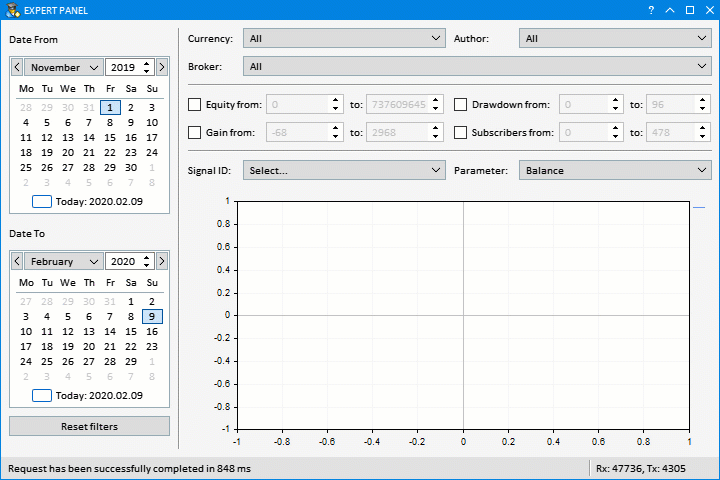Fig. 6. The program for viewing signal property dynamics in action

### Conclusion

In this article, we have considered the examples of applying the previously considered MySQL connector. While implementing the tasks, we have discovered that using the constant connection during frequent queries to the database is the most reasonable solution. We have also highlighted the importance of a ping for preventing the connection termination from the server side.

As for the network functions, working with MySQL is only a small part of what can be implemented with their help without resorting to dynamic libraries. We live in the age of network technologies, and the addition of the Socket function group is undoubtedly a significant milestone in the MQL5 language development.

The attached archive contents:

• Services\signals_to_db.mq5 — the source code of the data collection service
• Experts\signals_from_db\ — the source codes of the program for viewing signal property dynamics
• Include\MySQL\ — MySQL connector source codes
• Include\EasyAndFastGUI\the library for creating graphical interfaces (as of the date of posting the article)

Translated from Russian by MetaQuotes Software Corp.
Original article: https://www.mql5.com/ru/articles/7495

Attached files |
MQL5.zip (355.92 KB)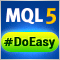Library for easy and quick development of MetaTrader programs (part XXXIV): Pending trading requests - removing and modifying orders and positions under certain conditions

In this article, we will complete the description of the pending request trading concept and create the functionality for removing pending orders, as well as modifying orders and positions under certain conditions. Thus, we are going to have the entire functionality enabling us to develop simple custom strategies, or rather EA behavior logic activated upon user-defined conditions.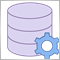Applying network functions, or MySQL without DLL: Part I - Connector

MetaTrader 5 has received network functions recently. This opened up great opportunities for programmers developing products for the Market. Now they can implement things that required dynamic libraries before. In this article, we will consider them using the implementation of the MySQL as an example.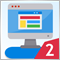Multicurrency monitoring of trading signals (Part 2): Implementation of the visual part of the application

In the previous article, we created the application framework, which we will use as the basis for all further work. In this part, we will proceed with the development: we will create the visual part of the application and will configure basic interaction of interface elements.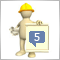Auto-Generated Documentation for MQL5 Code

Most Java coders will be familiar with the auto-generated documentation that can be created with JavaDocs. The idea is to add comments into the code in a semi-structured way that can then be extracted into an easy to navigate help file. The C++ world also has a number of documentation auto-generators, with Microsoft's SandCastle and Doxygen being two leaders. The article describes the use of Doxygen to create HTML help file from structured comments in MQL5 code. The experiment worked very well and I believe the help documentation that Doxygen produces from MQL5 code will add a great deal of value.